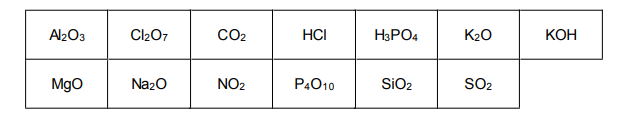# IMAT 2014 Q50 [Compounds]

How many of the following compounds are acidic, alkaline or amphoteric (react with both acids and alkalis)?A. Acidic = 10; Amphoteric = 2; Alkaline = 4
B. Acidic = 7; Amphoteric = 1; Alkaline = 5
C. Acidic = 9; Amphoteric = 2; Alkaline = 2
D. Acidic = 6; Amphoteric = 1; Alkaline = 6
E. Acidic = 8; Amphoteric = 1; Alkaline = 4